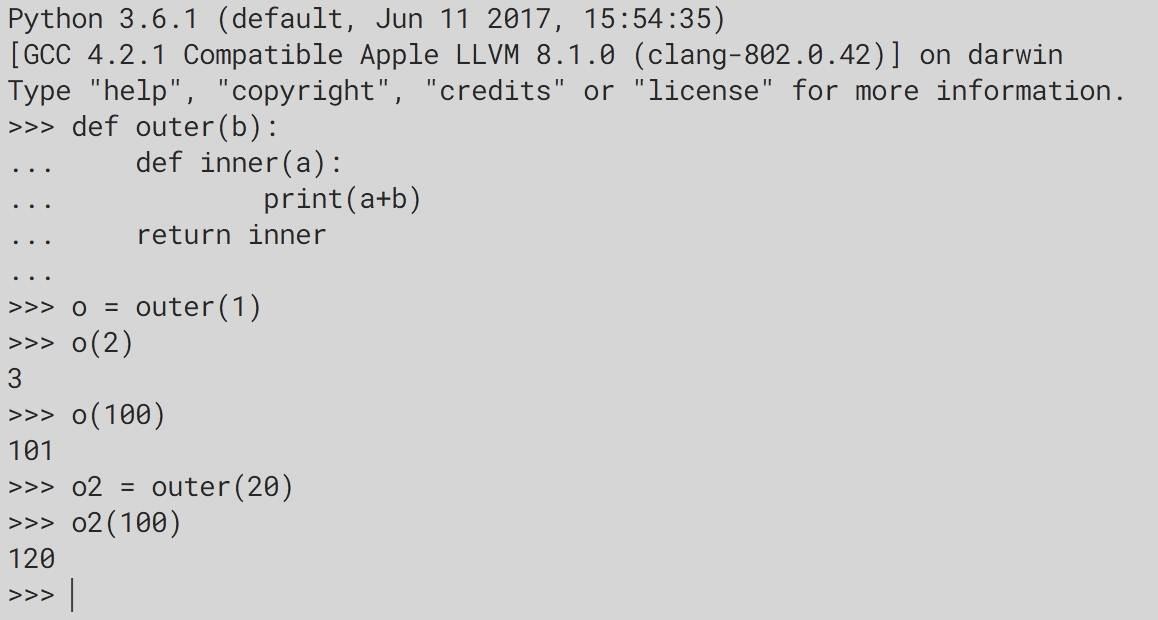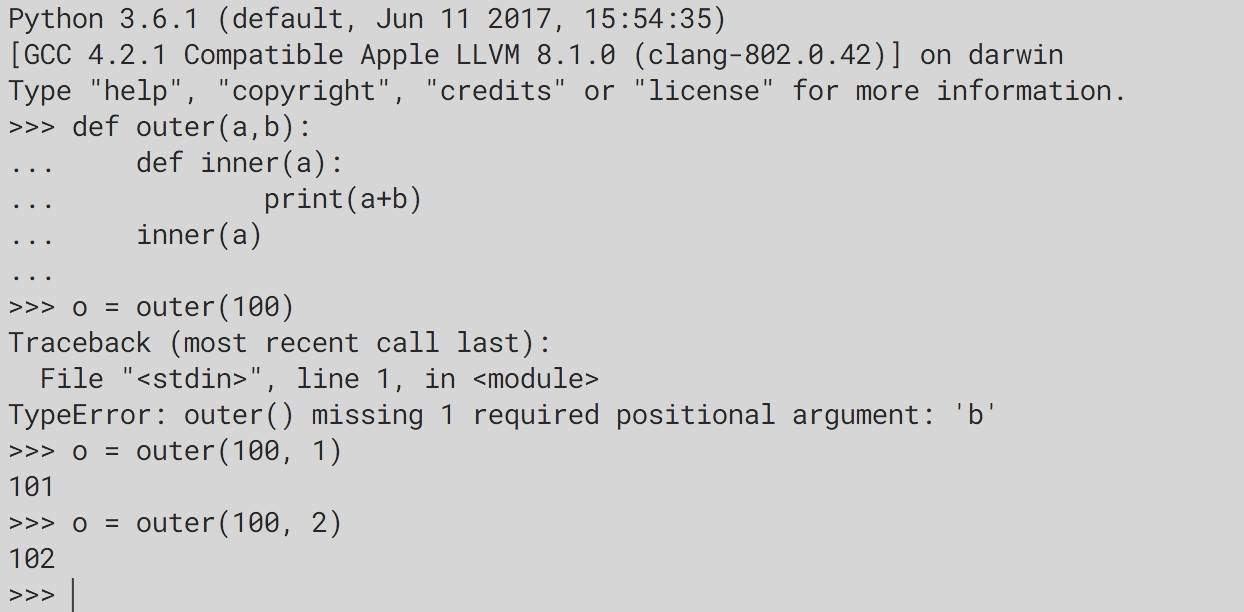# 简简单单说个闭包

## 闭包的条件

1. 外函数中定义了一个内函数
2. 内函数用了外函数的变量
3. 外函数返回了内函数的引用，or，外函数中直接调用了内函数

P.S.

1. 其中外函数和内函数是指嵌套函数中外部函数和内部函数
2. 也正是因为需要嵌套函数，因此不支持的嵌套函数的语言也自然不支持此类闭包
3. 条件3中分成了两类，更多的情况下是前一类，而后一类（外函数直接调用了内函数）的使用更多的是为了保证代码的简洁，而如此地保持简洁并不一定用闭包。

## 闭包的例子

“Talk is cheap, show me your code.”

``````def outer(b):
def inner(a):  # 条件1
print(a + b)  # 条件2
return inner  # 条件3

# 调用
o = outer(1)
o(2)
``````

Python的代码还是挺简单的。o和o2看起来就有那么一丝熟悉的感觉，它们两个就像是两个对象 —— 这两个“对象”都是从同一个“类”出来的，而两个“对象实例”的区别是有一个加数不一样，分别是1和20（当然，这两个变量的引用地址也不同）。

``````def outer(a, b):
def inner(a):  # 条件1
print(a + b)  # 条件2
inner(b)  # 条件3

# 调用
o = outer(100，1)
````````````func outer(i int) func() int {
return func() int {  // 条件1（匿名）+ 条件3
i++  // 条件2
return i
}
}

// 调用
o := outer(1)
o()
``````

``````function outer(\$str1) {
\$outerStr = \$str1;
\$inner = function(\$str2) {  // 条件1
echo \$str2 . \$outerStr;  // 条件2
};
return \$inner;  // 条件3
}

// 调用
\$o = outer("hahaha");
\$o("emmm");
``````

## 闭包的原因

### 闭包保存了函数的状态信息

``````def outer(b):
def inner(a):
print(a + b)
return inner

o = outer(1)
o(2)  # 3
o(100)  # 101
o2 = outer(20)
o2(100)  # 120
``````

o这个变量对应的闭包保存了`b=1`这个信息，之后无论是调用`o(2)`还是`o(100)``b=1`这个信息依然会存在并和后来的参数一起参与运算。同理，o2这个变量对应的闭包保存了`b=20`这个信息。

### 语法糖

``````# 先实现一个类似于装饰器的函数
def decorator(func):
def inner():
print 'before function'
func()  # function
print 'after function'
return inner

# 实现一个假装在登录的登录函数

# 将登录函数“套上”装饰器
``````

``````def operator(o):
def plus(x, y):
print(x + y)
def minus(x, y):
print(x - y)

if o == '+':
return plus
if o == '-':
return minus

def f(x, o, y):
operator(o)(x, y)
``````

## 总结

### 1 Comment